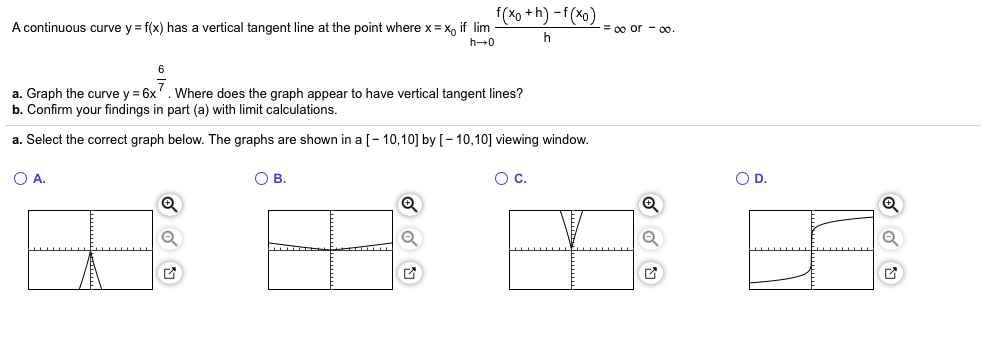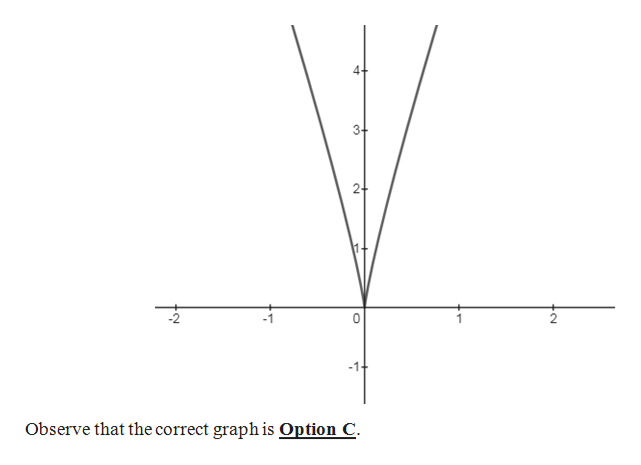f(xo+h)-f(xo)A continuous curve y f(x) has a vertical tangent line at the point where x x if lim= 00 or-00h 0a. Graph the curve y 6xb. Confirm your findings in part (a) with limit calculationsWhere does the graph appear to have vertical tangent lines?a. Select the correct graph below. The graphs are shown in a [- 10,10] by [- 10,10] viewing window.O D.O A.О в.Ос.

Question

Can you please help me step by step with this problem?help_outlineImage Transcriptionclosef(xo+h)-f(xo) A continuous curve y f(x) has a vertical tangent line at the point where x x if lim = 00 or-00 h 0 a. Graph the curve y 6x b. Confirm your findings in part (a) with limit calculations Where does the graph appear to have vertical tangent lines? a. Select the correct graph below. The graphs are shown in a [- 10,10] by [- 10,10] viewing window. O D. O A. О в. Ос. fullscreen
Step 1

(a) The graph of the given fu...help_outlineImage Transcriptionclose2+ Observe that the correct graph is Option C C fullscreen

Want to see the full answer?

See Solution

Want to see this answer and more?

Our solutions are written by experts, many with advanced degrees, and available 24/7

See Solution
Tagged in

Limits